[BACK]
 Intelligent Automation & Soft ComputingDOI:10.32604/iasc.2022.025127Article

Optimal and Energy Effective Power Allocation Using Multi-Scale Resource GOA-DC-EM in DAS

*Corresponding Author: J. Rajalakshmi. Email: lakshmijeyapal@gmail.com
Received: 12 November 2021; Accepted: 10 January 2022

Abstract: Recently many algorithms for allocation of power approaches have been suggested to increase the Energy Efficiency (EE) and Spectral Efficiency (EE) in the Distributed Antenna System (DAS). In addition, the method of conservation developed for the allocation of power is challenging for the enhancement because of their high complication during estimation. With the intention of increasing the EE and SE, the optimization of allocation of power is done on the basis of capacity of the antenna. The main goal is for the optimization of the power allocation to improve the spectral and energy efficiency with the increased capacity of the cable with the help of an efficient optimal method with the model of clustering. In order to attain optimized allocation of power and for antenna optimization, the algorithm of Multi-scale Resource Grasshopper Optimization (MSR-GOA) is implemented. Besides, the clustering process is carried out using the algorithm for clustering namely Discriminative cluster-based Expectation Maximization (DC-EM) so as to minimize the rate of interference and computing complication. The analysis of performance is employed for evaluating the projected performance in various scenarios. The existing approach of investigation and comparison is made with the suggested system (DAS with MSR-GOA-DC-EM) with respect to EE and SE. From the analysis, it was apparent that the method projected here is highly efficient to provide high spectral and energy efficiency than the already available techniques.

Keywords: DAS; multi scale resource grasshopper optimization algorithm; power allocation; spectral efficiency; energy efficiency; discriminative cluster-based expectation maximization

1  Introduction

Recently, there was a considerable increase in the cellular network’s data traffic. The development in the system of data leads to the higher requirements on the cellular system infrastructure. Consequently, this is declared as an imperative concern for passing on the effectiveness to cellular systems . A lot of researchers have presented distributed antenna systems (DAS) which in turn decreases the average distance for accessing among the User Equipment’s (UE) and the Remote Access Units (RAUs) to achieve the higher rate of data. This DAS is regarded to be the potential method in green contracting to improve the volume and to augment the Energy Efficiency [2,3]. Recently, EE has concerned substantial concentration because of the utilization of the growing energy exponentially in the communication or network using wireless. Besides wireless communication with energy efficiency recognized in the form green communication which has turned out to be a conventional one for the future wireless communication [4,5]. In the meantime, DAS was considered to be the key technology in green communication meant to increase the coverage as well as for the enhancement of the energy efficiency EE .

To resolve the problem of power allocation several existing models were presented in the field of DAS communication. The time requirement for computing the allocation of power policy is very large. This is not reasonable for the users in real life. So as to evade this issue there is a need to identify a good solution to enhance the speed and to reduce the complication of computation. Nowadays several effective applications have been attained by means of machine learning technologies in wireless communication. So as to raise the SE and EE the research based on designing the actual power allocation strategy was considered as a hotspot always. The optimization algorithm should be employed for getting better efficiency. In this approach multi-scale resource grasshopper algorithm is utilized. Base station, number of users in the base stations (BS) and the antenna are taken into consideration in this study. An effective way for sharing resources to both nearest user and distant user equivalently is concerned. The efficiency which is allocated should be offered to the users without considering the distance.

The main aim of this work is mentioned below as: increasing the EE and SE, the optimization of allocation of power is done on the basis of capacity of the antenna. In order to achieve optimized allocation of power and for the antenna optimization, MSR-GOA is developed. The clustering process is carried out using the algorithm for clustering, namely DC-EM so as to minimize the rate of interference and computing complication in a significant manner. Power allocation in the Distributed Antenna System should be optimal along with the rise in SE and EE.

The residual portion of the manuscript is ordered as shown. Section 2 is the deliberation of different existing approaches presented so far related to DAS. Section 3 is the detailed narration of the proposed strategy. The analysis of performance and the analysis of comparison of the suggested scheme is illustrated in section 4. At last, the conclusion covers the overall work strategy.

2  Related Works

The brief narration on the various available techniques associated with the allocation of power strategy and distributed antenna system is provided in this section.

The author in  examined the large perspective of association of machine learning technology and wireless communications. In this method, the intention of machine learning was taken into account for the Distributed Antenna System that was highly practical having low complexity of computation.  presented a scheme of EE allocation of power on behalf of the downlink many users DAS. The intention was to enhance the EE in the constraint on relative data rates and transmission of power for per antenna.  informed two various optimization issues planned for Distributed Antenna System and D2D communication. The main issue was increasing the spectral efficiency of Distributed Antenna System with the support of D2D communication in a lower SE constraints of D2D and DAS pair, D2D highest transmit power of the transmitter and each Remote Access Unit highest power of transmission.  suggested a scheme of most reasonable allocation of power for DAS with Orthogonal Frequency Division multiplexing (DAS-OFDM) in case of excessive frequency selection channel fading.  initiated a new approach intended for integration of DAS and non-orthogonal multi access. A number of novel techniques related to suboptimal allocation of power were presented. They were revealed to yield a secure application to the optimal allocation of power.  presented a md (massively dense) DAS along with the help of Virtual Cells. An energy efficiency related allocation of power policy was implemented in this method. The interference of inter Virtual Cells is taken into the mdDAS downlink. Thus the issue could be put together as a convoluted problem of fractional non convex programming.  discussed three different objectives for optimization of Distributed Antenna System. The sum rate is enhanced in the requirement of the system associated with lower spectral efficiency conditions and the Remote Access Unit power of transmission completely. The power of transmission is reduced and the reduction in spectral efficiency of system needs and every Remote Access Unit power of transmission entirely. For improving energy efficiency and satisfying the lower needs of spectral efficiency of the system and the overall transmission power of Remote Access Unit.  projected an aim of increasing the ergodic secrecy rate using the optimization of power allocation for each and every Remote Antenna in the condition of power of per antenna.  aimed at solving the maximization of Energy Efficiency complexity for sending many users in a Multiple Input Single Output (MISO) DAS.  recognized a communication of mm-wave as a candidate method. Distributed Antenna System consists of a Base Station BS which is connected to several Remote Radio Units RRU declared to enhance the energy efficiency.  examined the issues associated to various optimization of allocation of power along with the interferences of Distributed Antenna System with and lack of user centric method of Virtual Cells correspondingly.  suggested antenna packed together with 3d resolution to produce automotive parts. Wireless LAN, LTE and GPS are included in the solution of antenna, in the WAVE Wireless Access Vehicular Environment correspondingly.  intended to increase the EE that was mentioned as ratio of rate of transmission for the consumption of total power to the highest power transmission for the requirement of remote antenna and Quality of Service constraint.  introduced EE enhancement scheme that was defined by the mean rate ratio of the transmission was defined as ratio of mean rate of transmission to the sum of power consumption. Depending on this, a scheme of optimal Energy efficiency Power Allocation was improved for the DAS by choosing antennas over many fading channels.  investigated the enhancement of Energy efficiency issues in the DAS along with a laid communication of device-to-device. The optimization of EE depends on the CSI (Channel State Information) along with D2D pairs and Cellular UE (User Equipments) interference.  presented a method of optimization in the transmission covariance to increase EE for only one user Distributed Antenna System, wherever the user and Remote Access Unit were together armed with the many antennas. Unlike preceding works, the rate of requirements and the choosing of Remote Access Unit were considered. There were several techniques employed so far, however there is a need to overcome the issues addressed in existing techniques like reduced spectral and energy efficiency. This is made possible by the implementation of some effective approach.

3  Proposed Work

The proposed mechanism function with their overall flow is deliberated in a detailed manner in this section. Initially, the initialization of parameters is made by the generation of a system model on considering the cellular user’s number with their locations. After that, the process of optimization is carried with the use of MSR-GOA technique at which the multiple objective function is thus estimated on the updation of individual grasshopper’s position. Based on the closeness probability degree and the Gaussian probability, the multi-objective function is evaluated. The best position will be reached once the population size exceeds the maximum number of iterations and thereby the present position will be declared as the old best position. If there is failure in this condition, then the process will get repeated till the condition is satisfied. Lastly, the process of clustering is carried from this optimized outcome with the use of the DC-EM algorithm at which the computation of log likelihood is carried on the evaluation of posterior probability. Finally, the prediction of suitable unique BS serving is done and the allocation of subcarrier power is carried with the use of Lagrange Multiplier. From this, the capacity of the antenna and energy efficiency (EE) are enhanced. The performance was estimated for the proposed system which is then compared with existing technologies to prove the effectiveness of the proposed strategy.

A. Initialization of parameter

Information about the base station, no of users, and the cellular radius at which the users are surrounded are initialized at this step. Depending on the radius, the radius r will be taken as 1000, with no base station as 5 with 50 number of users. Also, the minimal requirement of spectral efficiency, noise power, optical fiber power, static and dynamic power, path loss exponent α, drain efficiency and transmitter’s maximum power were too initialized. These constraints are regarded as the system model having specified radius with coordinates x and y.

A DAS downlink scenario is being considered in this approach. There exists M number of remotes access units (RAUs) that have one antenna and L number of cellular UEs together with single antenna structure in DAS. The deployment of M numbers of RAUs are made in the cell uniformly and are correlated to the central base station (BS). After that, the L number of UEs are randomly distributed in the cell and it is represented in Fig. 1 given below. Likewise, hm,l is being employed for representing the channel’s frequency response among mth RAU and lth UE that encompasses of huge-scale and small-scale fading and is expressed as shown:

Hm,l= gm,lwm,l (1)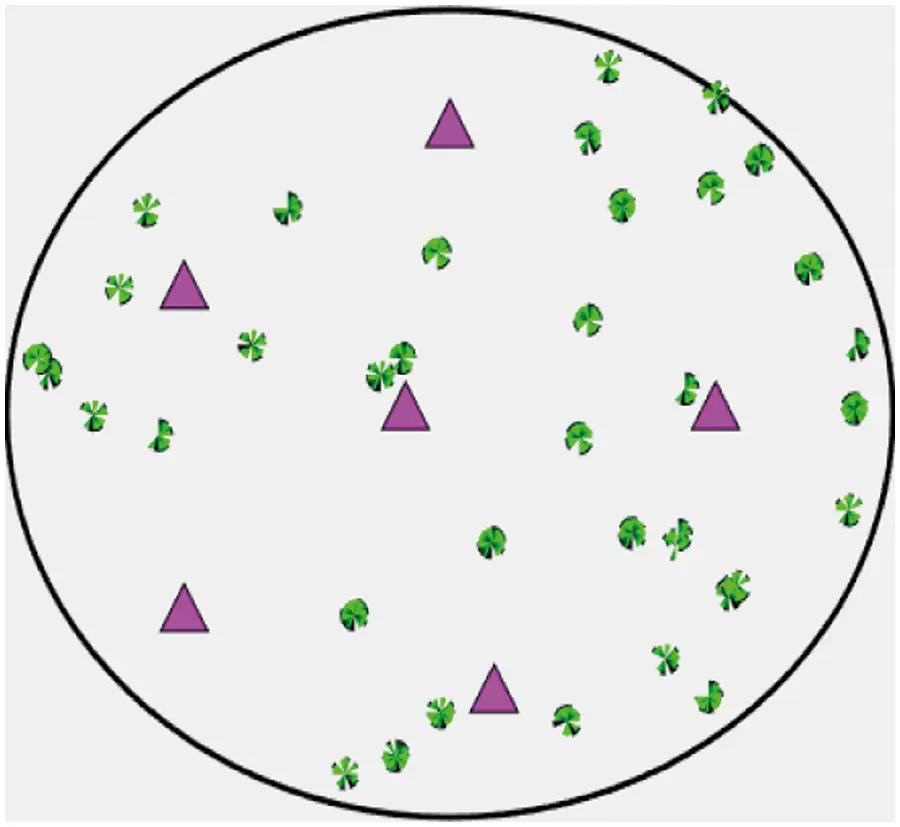Figure 1: System model

Here,

wm,l denotes a large-scale fading and is independent of gm,l.

gm,l denotes a small-scale fading among mth RAU and lth UE.

A representation of system model is provided in Fig. 1 as given below:

According to the coordinates created, the identification of distance is made by means of shadow fading by employing typical notation for distance estimation as follows:

Distance(d)=(x1y1)2+(x2y2)22 (2)

As per the values of distance estimated, the small-scale fading and mean power value is assessed. The estimation of channel gain based on the chosen coordinates x and y employed in acquiring large-scale fading values. As the initialization of parameter and configuration of the system is made, then the cellular user’s number along with its location is being updated. Then, the process of optimization is carried out with the use of MSR-GOA.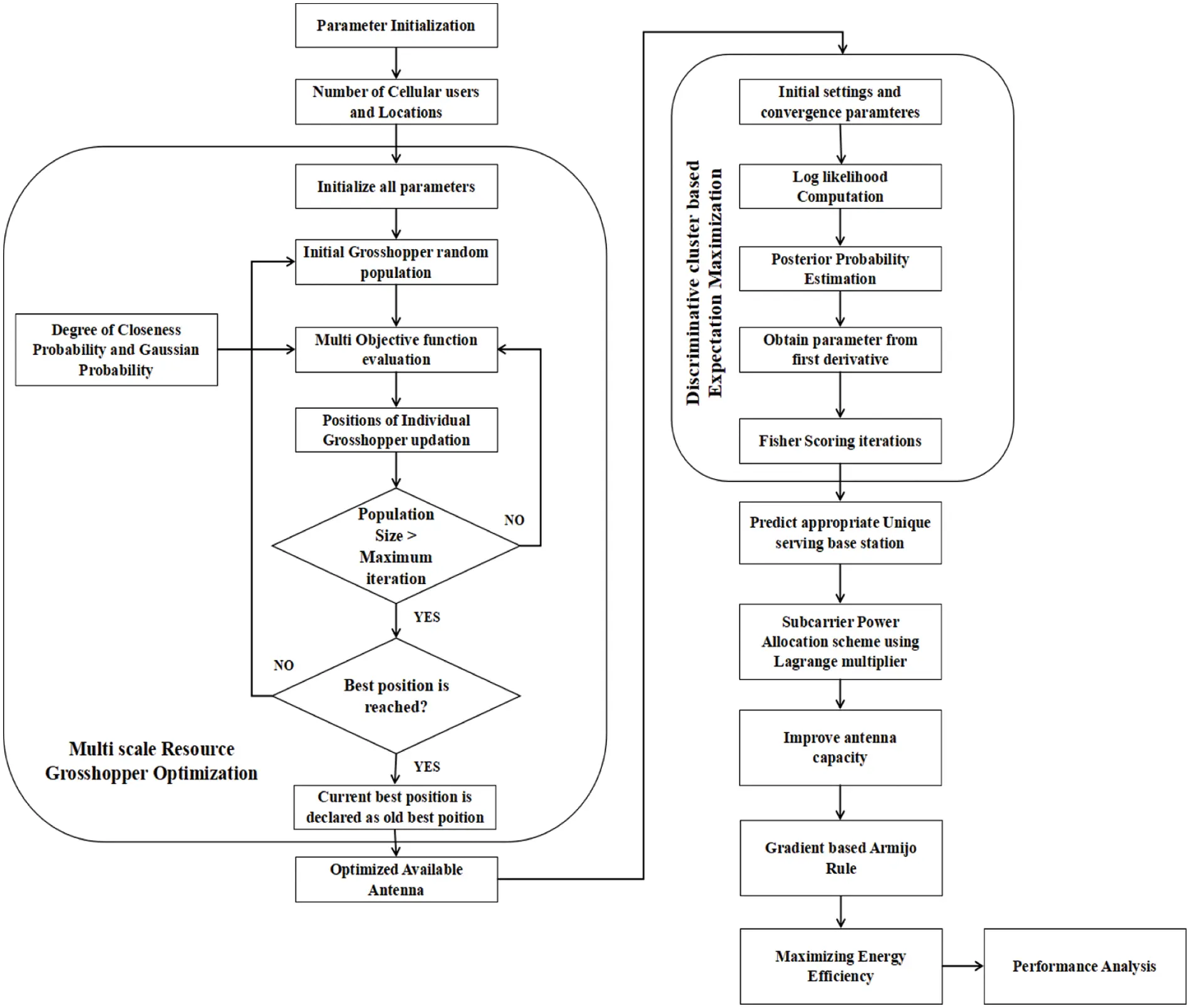Figure 2: Overall flow representation of proposed scheme

B. Multi-scale resource Grasshopper optimization (MSR-GOA)

Typically, the MSR-GOA algorithm has the ability to attain a better solution with some reasonable time frames. The proposed technique analysis includes convergence speed, exploitation ability and the ability of exploration.

In this optimization process, maximum iteration is taken as 1500. The parameters that are to be considered for this are as follows: number of search agents, maximum iteration, upper and lower bound dimension, search agent number and the distance assessed (X and Y coordinates, power and no of base stations). From this, the optimization method is carried out through the estimation of fitness function which thus determines the SE and EE enhancement. The evaluation of fitness function is carried for the estimation of optimal values at which the distance or closeness is identified for attaining enhanced efficiency. The best fitness function value computation is illustrated in the below provided algorithm. The optimization technique is carried out with the use of a MSR-GOA process. Once the initialization of parameters is made with random grasshoppers’ population, then based on the closeness probability degree and the Gaussian probability, the multi-objective function is evaluated. The best position will be reached once the population size exceeds the maximum number of iterations and thereby the present position will be declared as the old best position. If the condition is not satisfied, then the process gets repeated till it reaches the required condition. The algorithm for this process is illustrated below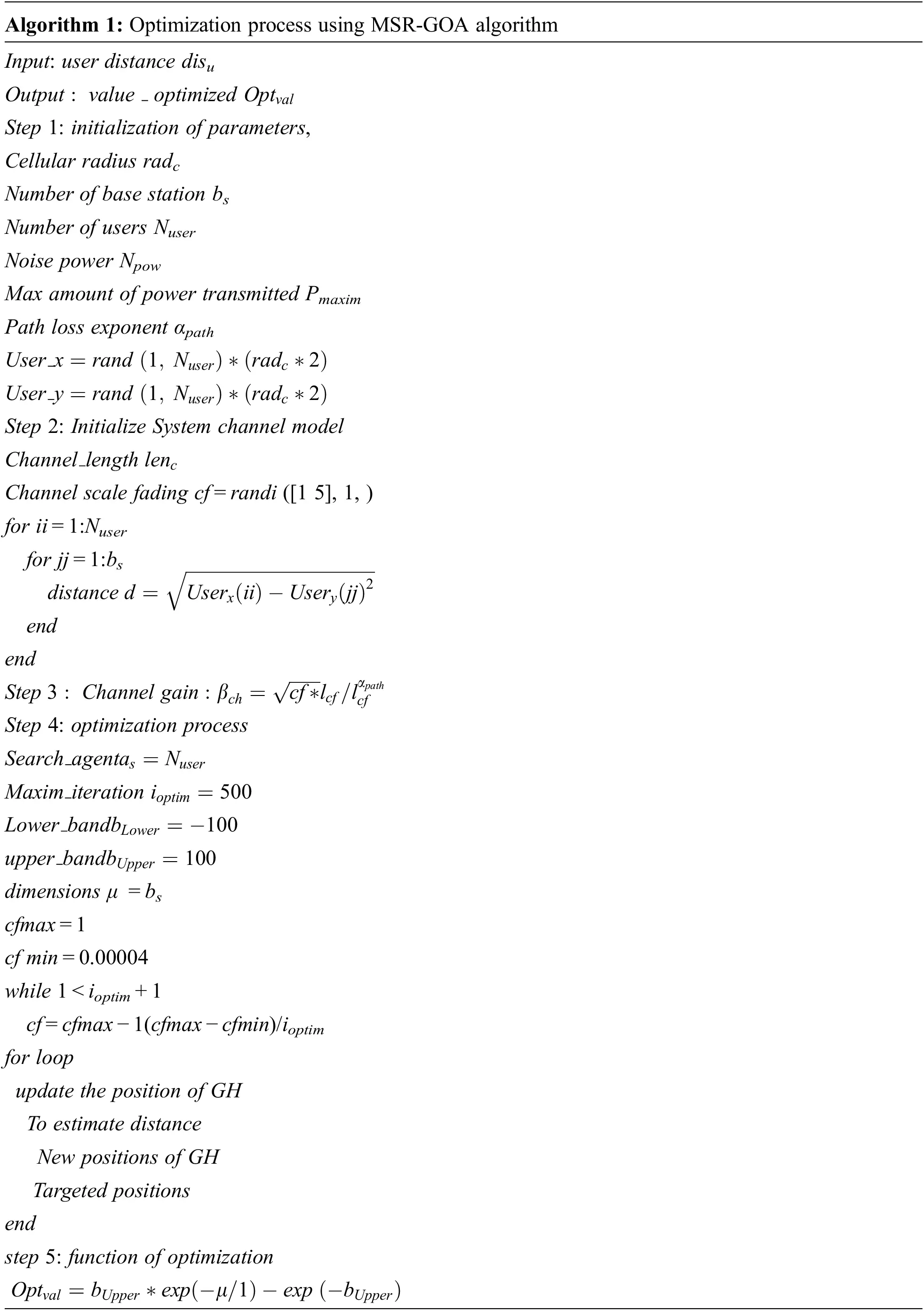In the approach of MSR-GOA, the distance user is regarded as input. At first, the parameters like no of base station, cellular radius, noise power, channel length, no of users, path loss exponent, and maximum transmitted power are initialized. The channel length is recognized in the system channel model using distance and channel scale fading values. Similarly, the channel gain is evaluated with the use of distance estimation. After that, in the process of optimization a search agent is regarded with a maximum number of iterations as 500 along with upper and lower bound dimensions as 100. The limit of upper and lower bound is being fixed and once the condition is satisfied the position of GH is thus updated on identifying the distance, global positions, and GH’s new position. At last, a best optimal fitness function along with optimal available antenna is obtained.

The optimized users gathered depending on resources are then gathered or clustered using the DC-EM process. The representation of trajectory 1st grasshopper is shown in Fig. 4.Figure 3: Users optimized depending on MSR-GOA approach presented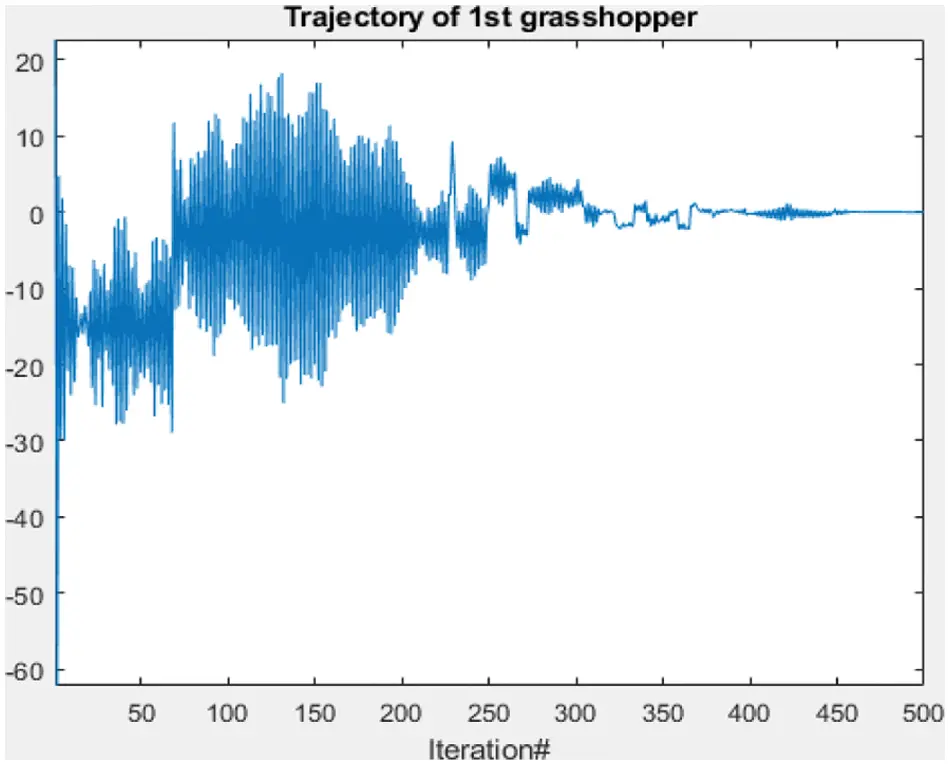Figure 4: Representation of trajectory of first grasshopper

C. Clustering process using DC-EM (Discriminative cluster-based Expectation Maximization)

Recently, the application of databases was increased so as to deal with huge datasets that are dimensional, thus this clustering process has emerged as an important process in wide research areas. Hence, a novel clustering approach termed DC-EM is presented for enhancing the strategy of power allocation. Typically, an EM algorithm is considered as an optimal way for recognizing maximum-likelihood estimates at which the data will be inadequate and contains unnoticed or hidden latent variables, or else has mislaid data points. It is considered as an iterative manner for the approximation of maximum likelihood function.

In the technique of proposed DC-EM, convergence parameters and initial setting are made which is then followed by the computation of Log Likelihood on assessing the posterior probability function from the initial derivative and the fisher scoring iterations. An algorithm for Discriminative cluster-based EM is illustrated as follows:By initializing the parameters of path loss exponent, static power and dynamic power the theory of EE is recognized which is followed by a number of iterations till the condition is satisfied for varying values of user path parameters. The process is carried till the maximum EE (Emax) is attained.

As per these iterative steps, the allocation of an optimal power along with maximum EE in DAS is thus obtained with the use of a projected approach. The clustered result attained by the DC-EM utilization is represented in Fig. 5.Figure 5: Outcome of cluster obtained using DC-EM

D. Strategy of allocating Subcarrier power and EE maximization

At last, the prediction of suitable unique BS serving is done and the allocation of subcarrier power is carried with the use of Lagrange Multiplier. From this, the capacity of the antenna and energy efficiency (EE) are enhanced. Then, the gradient-based Armijo rule is carried for Energy and spectral efficiency maximization. An estimation of performance is made so as to validate the proposed system effectiveness and thus to relate and compare the enhancement of efficiency with traditional techniques.

4  Results Analysis

At this presented approach, the allocation of power for the purpose of maximizing EE and SE is chosen. A numerical outcome was evaluated and offered for validating the presented algorithms efficiency by comparison of the proposed technique DAS with MSR-GOA-DC-EM with that of traditional techniques [4,22,23].

The analysis of EE with respect to number of UEs for number of base station antennas is represented in Fig. 6. Therefore, the attained fitness in average of entire grasshopper is represented in the graphical depiction. Moreover, Fig. 7 is the depiction of entire performance percentage of antenna.Figure 6: Average fitness of all grasshopper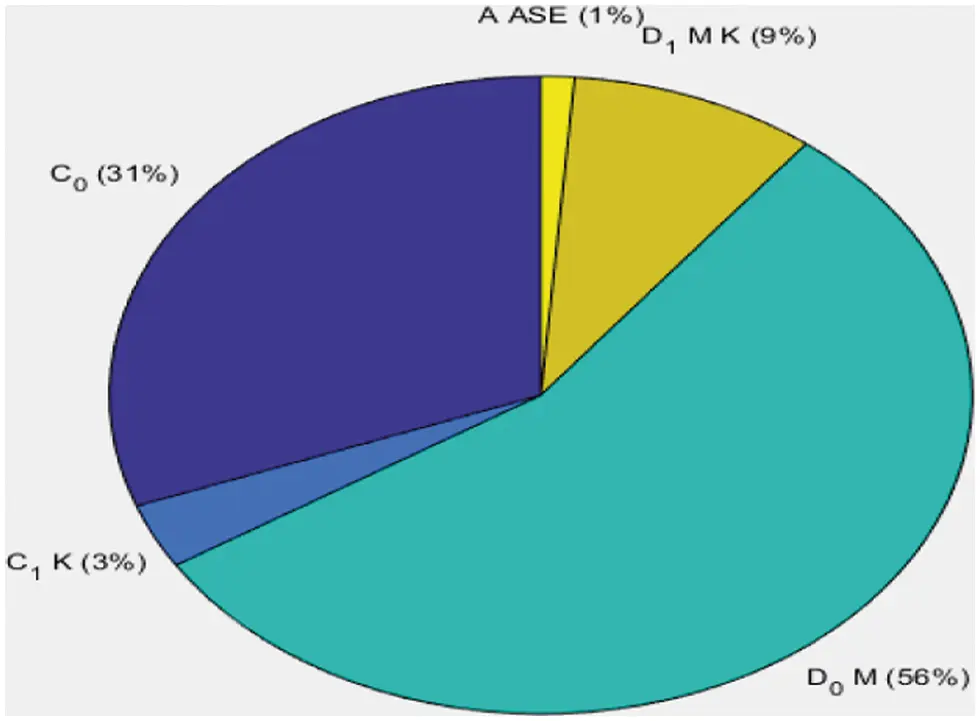Figure 7: Overall performance percentage of antenna

The estimation of performance was carried out in terms of EE for maximum transmit power with varying γ parameters range that is γ at 1, 3, and 7. The range of EE is denoted in Mbit/J. A graphical illustration of the outcome is shown in Fig. 8. The simulation parameter of the presented system is provided below in Tab. 1.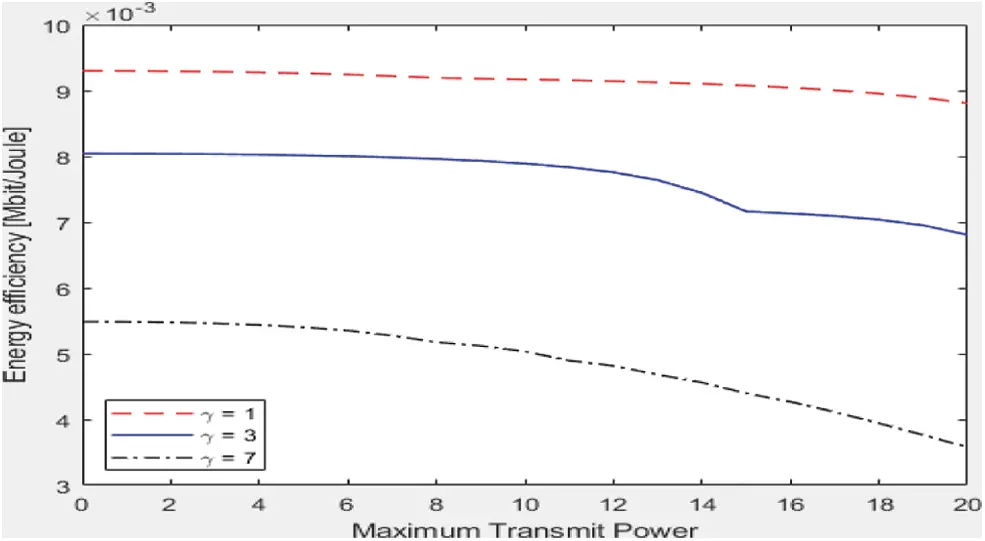Figure 8: Analysis of Energy Efficiency (EE) performance for varying γ parameterFigure 9: Spectral Efficiency (SE) comparative analysis (bits/Joule/Hz)Spectral Energy is estimated in terms of maximum transmit power at which the comparison of the presented technique is carried with that of the traditional KNN-SE approach. In this, the value of SE is mentioned in (bit/Joule/Hz). The analysis shows that the projected approach (DAS with MSR-GOA-DC-EM) is improved. A comparison was carried out among existing techniques KNN-SE and the projected DAS with MSR-GOA-DC-EM. An outcome reveals that the presented technique is far better in providing high EE on comparing the existing method. The depiction of comparison made for EE (bit/Hz) with respect to maximum transmit power (dBm) is shown below in Fig. 10.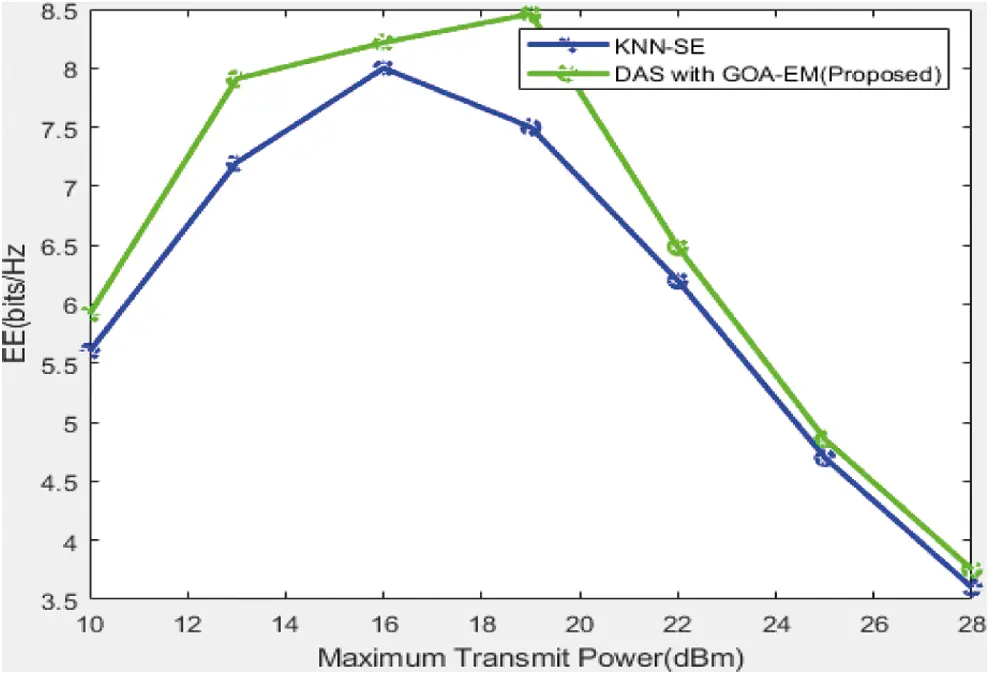Figure 10: Energy Efficiency (EE) comparative analysis (bits/Hz) 

The SE (bit/Hz) comparative analysis with respect to maximum transmit power is illustrated in Fig. 11. The comparison was carried out for conventional algorithms, DAS along with K-means cluster, and DAS with the mixture of Gaussian clustering technique , the SE of projected DAS with MSR-GOA-DC-EM is higher.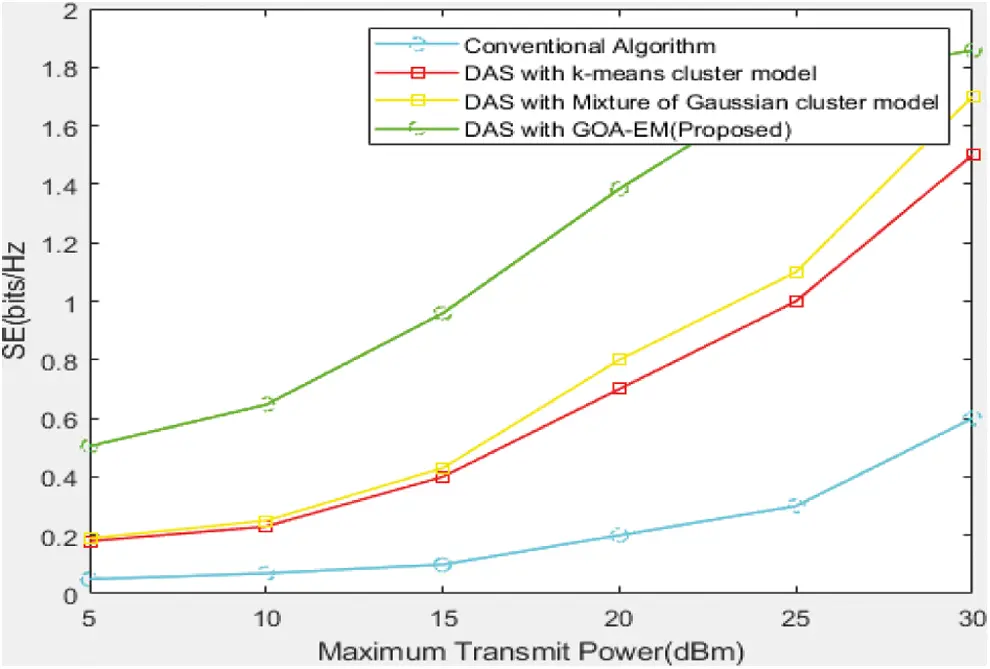Figure 11: Spectral Efficiency (SE) comparative analysis (bits/Hz) 

The comparison was carried out for conventional algorithms, DAS along with K-means cluster, and the DAS with the mixture of Gaussian clustering technique with the projected DAS with MSR-GOA-DC-EM. From the outcome it was evident that the projected strategy is better in offering high EE than the existing techniques. An EE (bit/joule/Hz) comparative analysis in terms of maximum transmit power (dBm) shown in Fig. 12.Figure 12: Energy Efficiency (EE) comparative analysis (bits/Joule/Hz) 

The study of comparison of Spectral Efficiency (bits/Hertz) on the basis of maximum transmit power dBm is illustrated in Fig. 13. The comparison was carried out for DAS with Device wise communication  and the suggested DAS with MSR-GOA-DC-EM algorithm. From the outcome it was obvious that the suggested strategy provides better high SE than the already existing methods. The comparison was carried out for DAS with Device-to-Device communication  and the suggested Distributed Antenna System DAS with MSR-GOA-DC-EM. From the analysis, it was obvious that the suggested strategy provides better high EE then the already existing methods.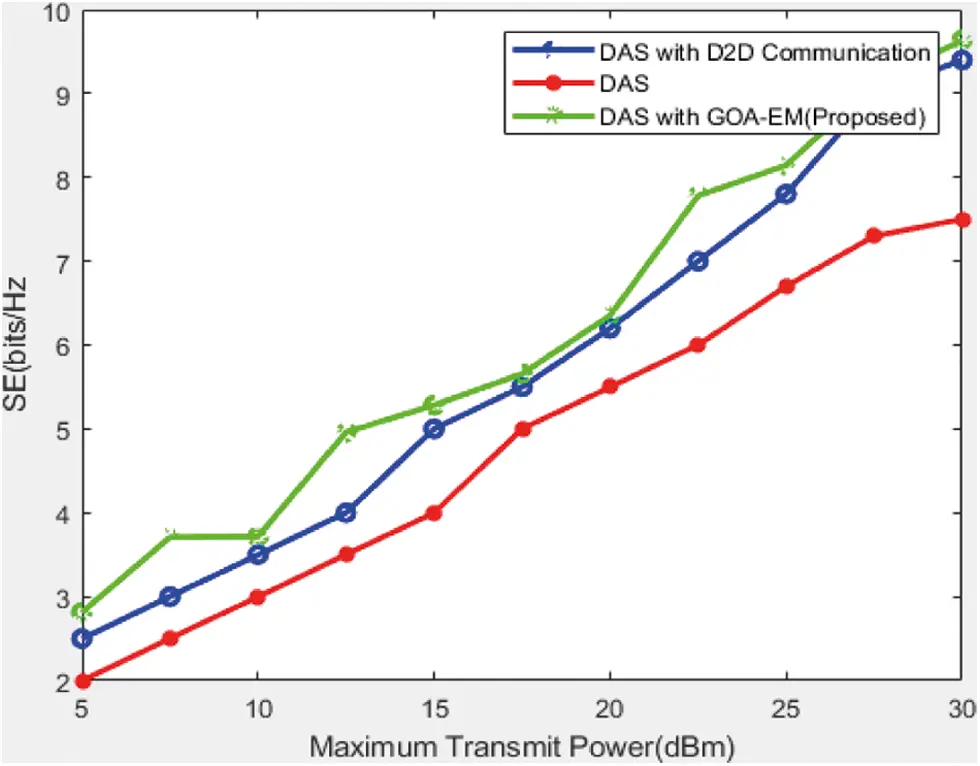Figure 13: Spectral Efficiency (SE) comparative analysis (bits/Hz) 

Fig. 14 depicts the study of comparison of Energy Efficiency (bit/joule/Hz) on the basis of maximum power of transmission dBm. In relation to the results of simulation, the performance is enhanced with the clusters mode in Distributed Antenna System.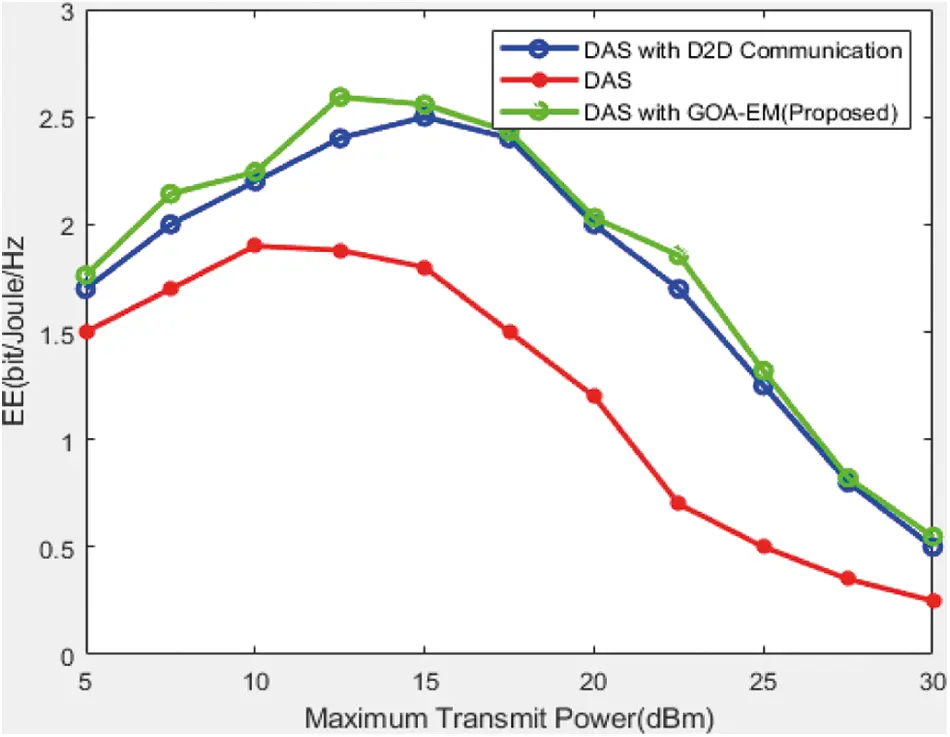Figure 14: Comparison of Energy Efficiency (EE) based on bits/Joule/Hertz 

In order to solve the complexities associated to maximize the EE and SE. As a result, the achieved power in the DAS with the support of the cluster’s mode was much more than the ancient scheme performed for allocating power. Thus, it makes the suggested scheme for the enhancement of EE and SE effective along with the minimized rate of computation of complexity.

5  Conclusion

The main aim concerned was the energy efficiency of power allocation in the Distributed Antenna System. The two main perspectives of this study are optimization and method of clustering. At first, with the support of MSR-GOA, optimization is done to attain the optimal capacity for antenna for the efficient power allocation and best operation for fitness. Then, the cluster method was created in the Distributed Antenna System with the help of DC-EM technique that was varied very much different from the traditional model of DAS. The enhancement of antenna capacity is performed using the subcarrier power allocation method using Lagrange multipliers. At last Armijo rule on the basis of the gradient is followed to increase the Energy Efficiency EE and spectral Efficiency SE. Several outcomes were provided and estimated for validating the effectiveness of applied algorithms by means of evaluating the suggested work (Distributed Antenna System with MSR-GOA-DC-EM) to that of the existing techniques. The outcome reveals that the proposed strategy is better in offering high EE and SE than the existing methods. In future, this work can be extended by employing deep learning-based methods.

Funding Statement: The authors received no specific funding for this study.

Conflicts of Interest: The authors declare that they have no conflicts of interest to report regarding the present study.

## References

1. G. Qian, Z. Li, C. He, X. Li and X. Ding, “Power allocation schemes based on deep learning for distributed antenna systems,” IEEE Access, vol. 8, pp. 31245–31253, 2020.
2. X. Yu, H. Wang, X. Wang, G. Wang and X. Dang, “Energy-efficient power allocation scheme for distributed antenna system over composite fading channels,” IEEE Access, vol. 6, pp. 18108–18116, 2018.
3. W. Xu, B. Wen, M. Lin and X. Yu, “Energy-efficient power allocation scheme for distributed antenna system over spatially correlated Rayleigh channels,” IET Communications, vol. 12, no. 5, pp. 533–542, 2017.
4. Y. Liu, C. He, X. Li, C. Zhang and C. Tian, “Power allocation schemes based on machine learning for distributed antenna systems,” IEEE Access, vol. 7, pp. 20577–20584, 2019.
5. W. Ying, Y. Xiangbin, W. Hao, C. Junya, D. Tao et al. “Adaptive energy efficient power allocation scheme for DAS with multiple receive antennas,” Transactions of Nanjing University of Aeronautics and Astronautics, vol. 35, no. 4, pp. 656–663, 2018.
6. J. Yan, H. Liu and Z. Bao, “Power allocation scheme for target tracking in clutter with multiple radar systems,” Signal Processing, vol. 144, pp. 453–458, 2018.
7. M. -L. Tham, S. F. Chien, D. W. Holtby and S. Alimov, “Energy-efficient power allocation for distributed antenna systems with proportional fairness,” IEEE Transactions on Green Communications and Networking, vol. 1, no. 2, pp. 145–157, 201
8. X. Li, C. He, D. Feng, C. Guo and J. Zhang, “Power allocation criteria for distributed antenna systems with D2D communication,” AEU-International Journal of Electronics and Communications, vol. 93, pp. 109–115, 201
9. X. Yu, H. Wang, S. -H. Leung and T. Teng, “Optimal power allocation scheme for distributed antenna system with OFDM in frequency-selective fading channels,” Electronics Letters, vol. 54, no. 7, pp. 454–456, 2018.
10. J. Farah, A. Kilzi, C. A. Nour and C. Douillard, “Power minimization in distributed antenna systems using non-orthogonal multiple access and mutual successive interference cancellation,” IEEE Transactions on Vehicular Technology, vol. 67, no. 12, pp. 11873–11885, 2018.
11. J. Wang, Y. Wang, W. Feng, X. Su and S. Zhou, “An iterative power allocation scheme for improving energy efficiency in massively dense distributed antenna systems,” in 2016 IEEE 83rd Vehicular Technology Conf. (VTC Spring), Nanjing, China, IEEE, pp. 1–5, 2016.
12. C. He, “Comparison of three different optimization objectives for distributed antenna systems,” AEU-International Journal of Electronics and Communications, vol. 70, no. 4, pp. 442–448, 2016.
13. H. -M. Wang, C. Wang, D. W. K. Ng, M. H. Lee and J. Xiao, “Artificial noise assisted secure transmission for distributed antenna systems,” IEEE Transactions on Signal Processing, vol. 64, no. 15, pp. 4050–4064, 2016.
14. H. Ren, N. Liu and C. Pan, “Energy efficient transmission for multicast services in MISO distributed antenna systems,” IEEE Communications Letters, vol. 20, no. 4, pp. 756–759, 2016.
15. L. Bai, T. Li, Q. Yu, J. Choi and W. Zhang, “Cooperative multiuser beamforming in mmWave distributed antenna systems,” IEEE Transactions on Vehicular Technology, vol. 67, no. 12, pp. 12394–12397, 2018.
16. X. Li, C. He and J. Zhang, “Spectral efficiency and energy efficiency of distributed antenna systems with virtual cells,” AEU-International Journal of Electronics and Communications, vol. 96, pp. 130–137, 2018.
17. O. -Y. Kwon, R. Song and B. -S. Kim, “A fully integrated shark-fin antenna for MIMO-LTE, GPS, WLAN, and WAVE applications,” IEEE Antennas and Wireless Propagation Letters, vol. 17, no. 4, pp. 600–603, 2018.
18. X. Yu, Y. Wang, Q. Zhu, T. Dong and J. Chu, “Energy efficient power allocation scheme for distributed antenna systems with target BER constraint,” China Communications, vol. 13, no. 11, pp. 234–243, 2016.
19. X. Dang, Y. Wang and X. Yu, “Suboptimal energy efficient power allocation scheme for distributed antenna system with antenna selection,” Electronics Letters, vol. 52, no. 4, pp. 264–266, 2016.
20. C. He, C. Tian, C. Zhang, D. Feng, C. Pan et al., “Energy efficiency optimization for distributed antenna systems with D2D communications under channel uncertainty,” IEEE Transactions on Green Communications and Networking, vol. 4, no. 4, pp. 1037–1047, 20
21. H. Ren, N. Liu, C. Pan and C. He, “Energy efficiency optimization for MIMO distributed antenna systems,” IEEE Transactions on Vehicular Technology, vol. 66, no. 3, pp. 2276–2288, 2016.
22. C. He, Y. Zhou, G. Qian, X. Li and D. Feng, “Energy efficient power allocation based on machine learning generated clusters for distributed antenna systems,” IEEE Access, vol. 7, pp. 59575–59584, 2019.
23. X. Li, C. He and J. Zhang, “Optimized power allocation scheme for distributed antenna systems with D2D communication,” IEICE Transactions on Communications, vol. 102, no. 5, pp. 1061–1068, 2019.This work is licensed under a Creative Commons Attribution 4.0 International License, which permits unrestricted use, distribution, and reproduction in any medium, provided the original work is properly cited.